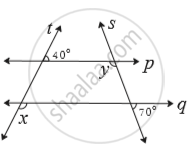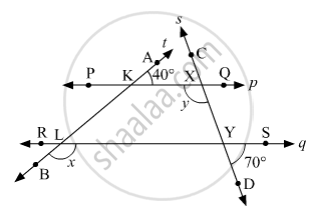# In the Adjoining Figure Line P || Line Q. Line T and Line S Are Transversals. Find Measure of ∠X and ∠Y Using the Measures of Angles Given in the Figure. - Mathematics

Sum

In the adjoining figure line p || line q.  Line t and line s are transversals. Find measure of ∠x and ∠y using the measures of angles given in the figure.#### SolutionLet us mark the points P and Q on p; R and S on q; A and B on t; C and D on s.
Suppose PQ and AB intersect at K; PQ and CD intersect at X.
Suppose RS and AB intersect at L; RS and CD intersect at Y.
Since, AB is a straight line and ray KQ stands on it,
m∠AKX + m∠XKL = 180°    (angles in linear pair)
⇒ 40° + m∠XKL = 180°
⇒ m∠XKL = 180° − 40°
⇒ m∠XKL = 140°
Since, p||q and t is a transversal, then
m∠YLB = m∠XKL    (Corresponding angles)
⇒ x = 140°
Since, RS and CD are two straight lines intersecting at Y, then
m∠XYL = m∠SYD    (Vertically opposite angles)
⇒ m∠XYL = 70°
Since, p||q and s is a transversal, then
m∠KXY + m∠XYL = 180°    (Interior angles on same side of transversal are supplementary)
⇒ y + 70° = 180°
⇒ y = 180° −  70°
⇒ y = 110°

Concept: Pairs of Lines - Transversal
Is there an error in this question or solution?

#### APPEARS IN

Balbharati Mathematics 8th Standard Maharashtra State Board
Chapter 2 Parallel lines and transversals
Practice Set 2.2 | Q 2 | Page 11